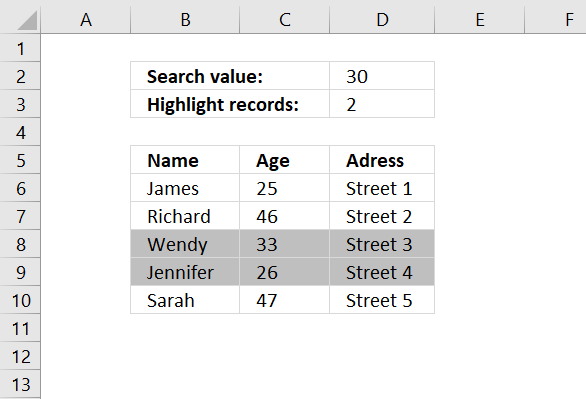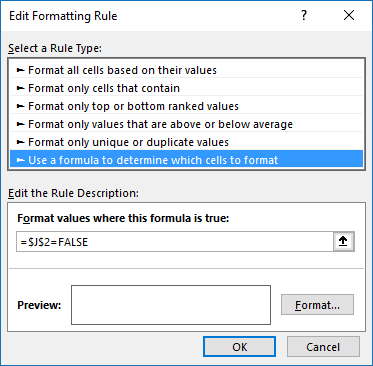Author: Oscar Cronquist Article last updated on January 11, 2019

###This post demonstrates how to highlight records with the closest value to a criterion, you can also choose to highlight the second closest value (or more).

You can also extract the number using the formula described in this article: Find closest value, perhaps you are also interested in how to find the numbers in a total closest to a given sum.

### Conditional formatting formula

=OR(ABS(\$C6-\$C\$2)=SMALL(ABS(\$C\$6:\$C\$10-\$C\$2), ROW(\$A\$1:INDEX(\$A:\$A, \$C\$3))))

This formula won't work in Excel 2010 and later Excel versions, simply create a named range containing the formula above. Then use that name as the conditional formatting formula, get the file later in this article if you need to see exactly how I did it.

### How to apply conditional formatting formula

1. Select cells B6:D10
2. Press with left mouse button on "Home" tab
3. Press with left mouse button on "Conditional Formatting" button
4. Press with left mouse button on "New Rule.."
5. Press with left mouse button on "Use a formula to determine which cells to format"
6. Type =OR(ABS(\$C6-\$C\$2)=SMALL(ABS(\$C\$6:\$C\$10-\$C\$2), ROW(\$A\$1:INDEX(\$A:\$A, \$C\$3)))) in "Format values where this formula is TRUE" window.(The formula displayed in the image above is not used in this article)
7. Press with left mouse button on "Format.." button
8. Press with left mouse button on "Fill" tab
9. Select a color for highlighting cells.
10. Press with left mouse button on "Ok"
11. Press with left mouse button on "Ok"
12. Press with left mouse button on "Ok"

### How the conditional formatting formula works in cell C6

=OR(ABS(\$C6-\$C\$2)=SMALL(ABS(\$C\$6:\$C\$10-\$C\$2), ROW(\$A\$1:INDEX(\$A:\$A, \$C\$3))))

Step 1 - Calculate age differences

=OR(ABS(\$C6-\$C\$2)=SMALL(ABS(\$C\$6:\$C\$10-\$C\$2), ROW(\$A\$1:INDEX(\$A:\$A, \$C\$3))))

ABS(number) returns the absolute value of a number, a number without its sign.

ABS(\$C\$6:\$C\$10-\$C\$2)

becomes

ABS({25;46;33;26;47}-30)

becomes

ABS({-5;16;3;-4;17})

and returns {5;16;3;4;17}

Step 2 - Create an array with the same size as chosen records to highlight in cell C3.

=OR(ABS(\$C6-\$C\$2)=SMALL(ABS(\$C\$6:\$C\$10-\$C\$2),ROW(\$A\$1:INDEX(\$A:\$A,\$C\$3))))

ROW(\$A\$1:INDEX(\$A:\$A,\$C\$3)))

becomes

ROW(\$A\$1:INDEX(\$A:\$A,2)))

becomes

ROW(\$A\$1:\$A\$2)

and returns {1;2}

Step 3 - Return the two smallest numbers in array

=OR(ABS(\$C6-\$C\$2)=SMALL(ABS(\$C\$6:\$C\$10-\$C\$2),ROW(\$A\$1:INDEX(\$A:\$A,\$C\$3))))

SMALL(array,k) returns the k-th smallest number in this data set.

SMALL(ABS(\$C\$6:\$C\$10-\$C\$2),ROW(\$A\$1:INDEX(\$A:\$A,\$C\$3)))

becomes

SMALL({5;16;3;4;17};{1;2})

and returns {3;4}

Step 4 - Is cell value in C6 equal to any number in array?

=OR(ABS(\$C6-\$C\$2)=SMALL(ABS(\$C\$6:\$C\$10-\$C\$2),ROW(\$A\$1:INDEX(\$A:\$A,\$C\$3))))

becomes

OR(logical1, logical2, ...) checks whether any of the arguments are TRUE, and returns TRUE or FALSE. Returns FALSE if only all arguments are FALSE.

\$C6 is a relative AND absolute cell reference.

=OR(ABS(\$C6-\$C\$2)={3;4})

becomes

=OR(ABS(25-30)={3;4})

becomes

=OR(ABS(-5)={3;4})

becomes

=OR(5={3;4})

becomes

=OR({5=3,5=4})

becomes

=OR({FALSE,FALSE})

and returns FALSE. Row 6 is not highlighted.

### Get the Excel fileHighlight-closest-values3.xlsx